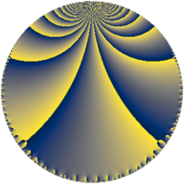# Properties

 Label 230.6.lLevel $230$ Weight $6$ Character orbit 230.l Rep. character $\chi_{230}(7,\cdot)$ Character field $\Q(\zeta_{44})$ Dimension $1200$ Sturm bound $216$

# Related objects

## Defining parameters

 Level: $$N$$ $$=$$ $$230 = 2 \cdot 5 \cdot 23$$ Weight: $$k$$ $$=$$ $$6$$ Character orbit: $$[\chi]$$ $$=$$ 230.l (of order $$44$$ and degree $$20$$) Character conductor: $$\operatorname{cond}(\chi)$$ $$=$$ $$115$$ Character field: $$\Q(\zeta_{44})$$ Sturm bound: $$216$$

## Dimensions

The following table gives the dimensions of various subspaces of $$M_{6}(230, [\chi])$$.

Total New Old
Modular forms 3680 1200 2480
Cusp forms 3520 1200 2320
Eisenstein series 160 0 160

## Trace form

 $$1200q - 8q^{3} + 160q^{6} + O(q^{10})$$ $$1200q - 8q^{3} + 160q^{6} - 128q^{12} + 2096q^{13} + 30720q^{16} + 20448q^{18} - 46880q^{23} + 9440q^{25} + 1760q^{26} + 4816q^{27} + 41536q^{28} + 11320q^{31} - 165044q^{33} - 25288q^{35} - 143360q^{36} + 144232q^{37} - 8520q^{41} - 51840q^{46} - 98480q^{47} + 2048q^{48} + 2848q^{50} + 33536q^{52} - 52480q^{55} + 14080q^{56} + 56232q^{57} + 500720q^{61} - 43072q^{62} - 630080q^{66} + 124704q^{70} - 1220880q^{71} - 72704q^{72} + 64312q^{73} - 698756q^{75} - 461704q^{77} - 100320q^{78} + 1582520q^{81} - 221280q^{82} - 430580q^{85} - 1266320q^{86} + 754760q^{87} - 100992q^{92} - 670504q^{93} + 1012292q^{95} - 40960q^{96} + 1165692q^{97} - 24096q^{98} + O(q^{100})$$

## Decomposition of $$S_{6}^{\mathrm{new}}(230, [\chi])$$ into newform subspaces

The newforms in this space have not yet been added to the LMFDB.

## Decomposition of $$S_{6}^{\mathrm{old}}(230, [\chi])$$ into lower level spaces

$$S_{6}^{\mathrm{old}}(230, [\chi]) \cong$$ $$S_{6}^{\mathrm{new}}(115, [\chi])$$$$^{\oplus 2}$$